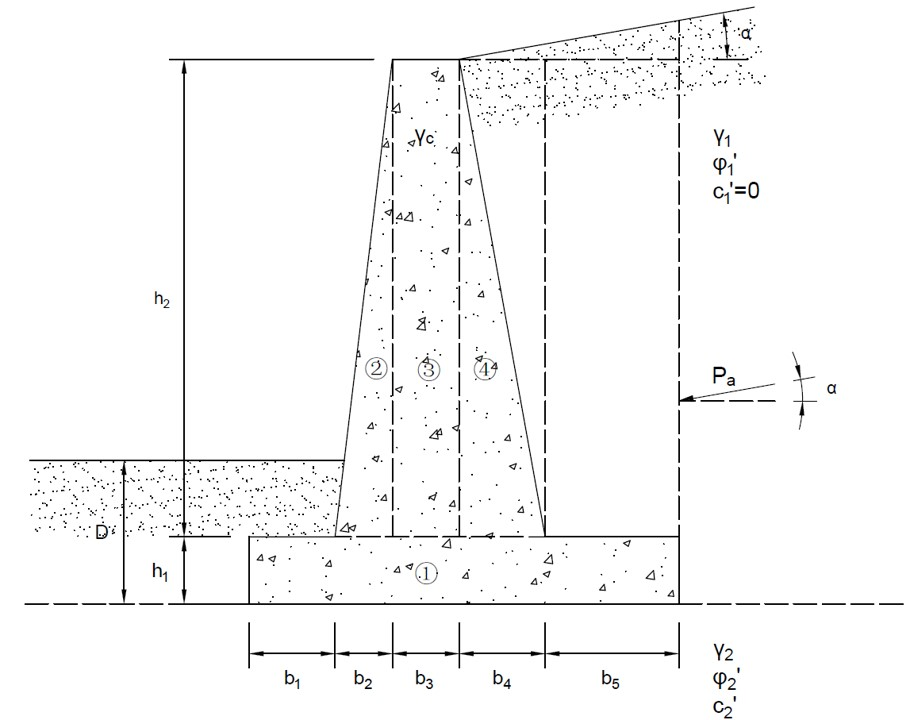# Retaining Wall Tool## Geometry of Retaining Wall## Tips

• This tool is for retaining wall analysis, as shown in the figure above.
• This tool is suitable for cantilever and gravity retaining walls using Rankine earth pressure theory.
• Two homogenous soil layers are considered in the analysis.
• The backfill layer is granular soil, and the cohesion c1 is assumed to be zero.
• The normal requirements for the stability check are listed as: FSO(Safety factor of overturning) > 2; FSS(Safety factor of base sliding) > 1.5; FSB(Safety factor of bearing capacity) > 3.
• All the input parameters should be positive.
• For "nan", "0" or "inf" displayed in Results, please check your input parameters.

D (m)
h1 (m)
h2 (m)
b1 (m)
b2 (m)
b3 (m)
b4 (m)
b5 (m)
α (°)
γc (kN/m3)
γ1 (kN/m3)
φ′1 (°)
γ2 (kN/m3)
φ′2 (°)
c2 (kN/m2)

## Output Results

• Rankine active pressure
Pa (kN/m) =
• Rankine passive pressure
Pp (kN/m) =
• Total vertical force acting on the base
ΣV (kN/m) =
• Maximum pressure under the base
qmax (kN/m2) =
• Minimum pressure under the base
qmin (kN/m2) =
• Ultimate bearing capacity
qu (kN/m2) =
• Safety factor of overturning
FSO =
• Safety factor of sliding
FSS =
• Safety factor of bearing capacity
FSBC =